# AREAS Function

Returns the number of areas that make up an MS Excel reference

## What is the AREAS Function?

The AREAS Function in Excel is an Excel Lookup/Reference function. This function will take an Excel reference and return the number of areas that make up the reference. It is available starting from MS Excel 2007.

### Formula

=AREAS(reference)

The AREAS function uses the following argument:

1. Reference (required argument) – This can be a standard Excel reference or a list of references or a named range.

### How to use the AREAS Function in Excel?

It is a built-in function that can be used as a worksheet function in Excel. To understand its uses, let’s consider a few examples:

#### Example 1

AREAS is a simple function of Excel.

Area = ranges or single cell

So, when we give the formula AREAS(B1), we get the result as 1.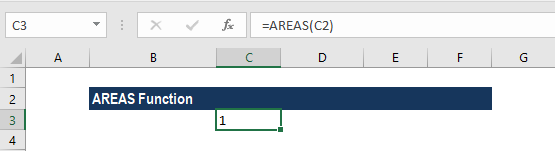Now let’s see how the function behaves when we provide ranges as a reference. As you can see in the screenshot below, we provided the formula for the highlighted range (yellow). The formula used is =AREAS(B6:B9).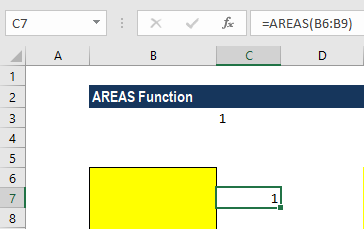This function will return 1, as the range selected is only one.

In our next example, two ranges are selected. The formula used, AREAS((B6:B9,B11:B15)), will give a result of 2. For selecting more than one range, we open a bracket and provide ranges separated by columns and close the brackets.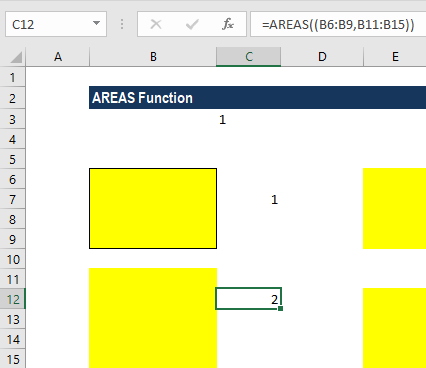On a similar basis, we will get a result of 3 when 3 ranges are selected, 4 when we select 4 ranges, and so forth. Remember to separate each range with a comma, or else it will give a #NAME? error.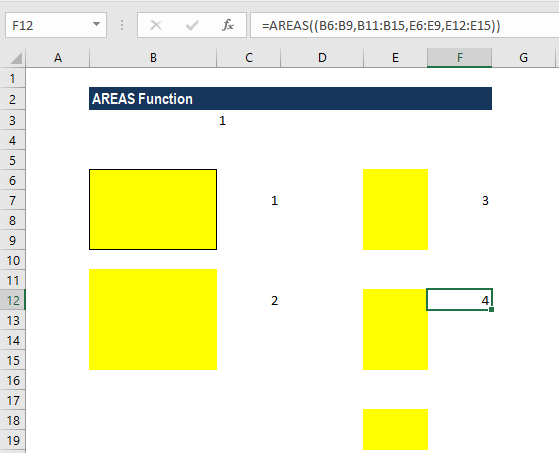AREAS is one of the less useful information functions in Excel. The function’s only use is to return the number of areas in a given range reference. Practically, there is little or no need for it at all, as it’s not required in those Excel functions that can handle general references such as SUM. Furthermore, it’s unnecessary for dealing with functions that can handle only single area ranges (like SUMIF) since we can use INDEX(Range,0,0,1) to refer to the first area of a general range reference.

#### Example 2

Suppose we’ve supplied the reference to a named range (called ‘E_Range’), which is made up of the 3 areas shown in the ‘Formula’ spreadsheet below.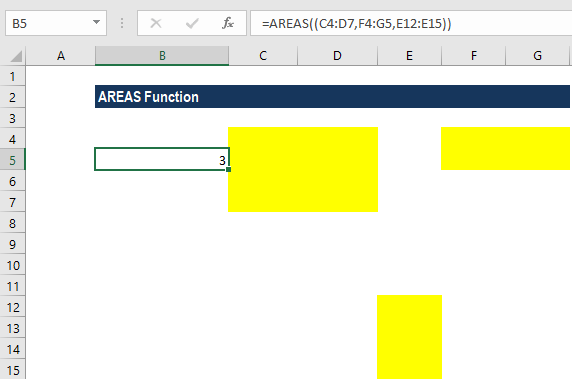In this scenario, the AREAS function returns the result 3.

### Things to remember in AREAS function

1. We can either type the entire formula or, after typing “=AREAS(“ into a cell, we can use the CTRL + A, without leaving the cell, to open the “Insert Function Dialog Box” for detailed commands.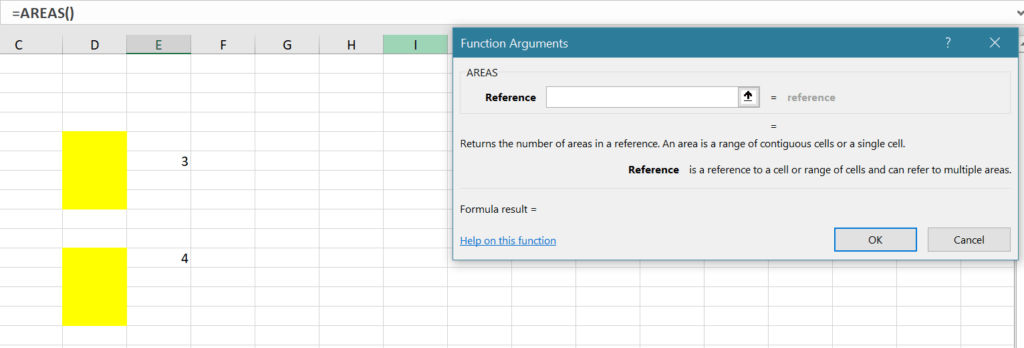1. A #NULL! error will be returned when the reference given is in an incorrect format. For example, AREAS(A2 B4) instead of AREAS(A2:A4).

Thanks for reading CFI’s guide to important Excel functions! By taking the time to learn and master these functions, you’ll significantly speed up your financial analysis. To learn more, check out these additional CFI resources:

• Excel Functions for Finance